Published: 30 June 2017

# Fault diagnosis of motorized spindle via modified empirical wavelet transform-kernel PCA and optimized support vector machine

Fei Chen1
Yifeng Ye2
Weizheng Chen3
Binbin Xu4
Chao Chen5
Zhaojun Yang6
1, 2, 3, 4, 5, 6School of Mechanical Science and Engineering, Jilin University, Jilin, China
Corresponding Author:
Chao Chen
Views 228

#### Abstract

The fault diagnosis of motorized spindle contributes to the improvement of the reliability of computer numerical control machine tools. Presently, numerous mechanical fault diagnosis technologies suffer from the drawbacks of mode mixing, non-adaptive analysis, and low efficiency. Therefore, adopting an effective signal processing method for fault diagnosis of motorized spindle is essential. A method based on modified empirical wavelet transform (EWT) and kernel principal component analysis (Kernel PCA) is proposed. A new method, which determines the proper number of the Fourier spectrum segments, is applied when using EWT. To improve computational efficiency, Kernel PCA is adopted to reduce dimension. The support vector machine optimized by genetic algorithm is introduced to accomplish fault identification. The performance of the proposed method is validated through single and compound fault experiments. Results show that the recognition rate using the proposed method reached 98.8095 % and 98.4375 % in terms of single and compound fault diagnoses, respectively. Moreover, compared with empirical mode decomposition (EMD), ensemble empirical mode decomposition (EEMD), local mean decomposition (LMD) and EWT, the proposed method can save much computing time. The proposed method can be generalized to other mechanical fault diagnoses as well.

## 1. Introduction

High-speed machining is a promising technology that drastically increases productivity and reduces production costs resulting in a revolutionary leap in manufacturing [1, 2]. The motorized spindle is crucial in implementing high-speed machining. And it is popular in manufacturing field because of its advantages, such as zero transmission, small vibration, precise control of rotational acceleration and deceleration [3, 4]. However, owing to the combination of tools and built-in motors, the motorized spindle is much more complex compared with the traditional spindle . The reliability of the motorized spindle remains to be improved mainly due to the complex structure which recently draw attention of industry and academy. This further indicates the importance of seeking methods for the fault diagnosis of the motorized spindle to improve the performance and reliability of computer numerical control (CNC) machine tools.

With the rapid development of signal processing technology, mechanical fault diagnosis technology has been intensively studied. Several approaches for mechanical fault diagnosis, including acoustic emission, oil monitoring, infrared temperature measurement, and vibration analysis, have been reported. Vibration analysis, which is extensively used in condition monitoring and fault diagnosis of mechanical equipment, is the most popular and effective approach [6-9]. The vibration signals of mechanical equipment include a large amount of information which is sensitive to different operation states. Therefore, signal processing techniques, especially in processing vibration signals, attract considerable interest over the past few years. However, using fast Fourier transform (FFT) for fault diagnosis present numerous problems in spectrum analysis, mainly owing to the vibration signals of mechanical equipment are non-linear and non-stationary, meanwhile, these signals are covered by a large scale of noise and closely spaced frequencies . To overcome these limitations, short-time Fourier transform (STFT) , Wigner-Ville distribution  and wavelet transform [13, 14] are widely applied as the most common time–frequency analysis tools. Some cases yielded desirable results with the use of the aforementioned methods for fault diagnosis. For example, a comparative study of FFT, STFT, and wavelet techniques for induction machine fault diagnostic analysis was performed by Mehala et al. . The experimental results show that STFT and wavelet transform provide better results than FFT and can effectively diagnose shorted turns and broken rotor bars in non-constant load torque induction-motor applications. Although STFT overcomes the shortcomings of FFT-based methods in processing non-linear and non-stationary signal, it cannot achieve low- and high-frequency components analysis simultaneously because its fixed spectral resolution provides the predefined window length, which inadequately describes instantaneous frequency [16-18]. Thus, STFT is suitable for processing quasi stationary signals instead of realistic non-linear and non-stationary signals . Wigner-Ville distribution has a high time and frequency resolution, but its application is restricted in multicomponent signal analysis because of cross-product term interferences [19, 20]. Compared with STFT, wavelet transform is a more effective tool in analyzing non-linear and non-stationary signals because of its property on multi-resolution analysis. Wavelet transform has already achieved good results in condition monitoring and fault diagnosis of mechanical equipment, such as bearing fault  and planetary gearbox fault detection . Dyadic wavelet transform is widely used in fault diagnosis of induction motors, rolling bearings, and other rotary machine owing to its high efficiency [23-25]. Unfortunately, as a non-adaptive algorithm, wavelet transform cannot decompose signals according to its contained information [26, 27], and a highly noisy environment immensely influences wavelet transform capability. What’s more, the analysis results using wavelet transform depend on the choice of predefined wavelet basis, which leads to a subjective and a priori assumption on the signal characteristics.

To solve shortages of wavelet, transform, namely non-adaptive and priori assumption, a completely different method named empirical mode decomposition (EMD) was proposed by Huang et al. . EMD gain fruitful achievements in various application fields since its introduction in 1998, such as seismic exploration, fault diagnosis, voice recognition, medicine, and biology. The basic principle of EMD is that practical signals are decomposed into a series of complete and almost orthogonal components called intrinsic mode function (IMF) [26, 28-30]. Each IMF is considered to be a mono-component, which indicates the natural mode contained in the original signal [9, 26]. In comparison with STFT, Wigner-Ville distribution and wavelet transform, EMD is more suitable for analyzing non-linear and non-stationary signals. Nevertheless, EMD is well known for the deficiency in the accurate estimation of instantaneous frequencies [26, 31]. For example, the EMD method cause end effects, and lacks mathematical theory basis, besides, mode mixing is found between IMFs, wherein the IMFs are not strictly orthogonal to each other [26, 32, 33]. Therefore, in order to decrease the effect of mode mixing, an improved version of EMD, namely ensemble empirical mode decomposition (EEMD) is proposed . The EEMD method is applied to rotor fault diagnosis of rotating machinery, and receive satisfactory results . Unfortunately, EEMD loses the advantage of adaptive decomposition, because it is still an open problem for adaptively selecting the amplitude of the added noise and determining the number of ensemble. In addition, many methods have been widely applied in the fault diagnosis of mechanical equipment, such as local mean decomposition (LMD), principal components analysis (PCA), independent components analysis (ICA), and EMD combined with other techniques. A method combining wavelet packet decomposition (WPD) and EMD was adopted to extract fault feature frequency, and a three-layered neural network for rotating machinery early fault diagnosis was proposed . The performance of the proposed method is illustrated through shaft fault experiment with lateral early crack of rotating machinery. For the intelligent fault diagnosis of induction motors, a method combined with ICA and support vector machines (SVM) was applied, and satisfactory results are obtained . The multi-variate and multi-scale monitoring of large-size low-speed bearings using EEMD combined with principal component analysis was executed . The proposed method can accurately identify the local bearing small defects. Han et al.  adopted LMD combined with sample entropy and energy ratio to process the vibration signals of roller bearings, and then, different operation states can be classified using SVM. Du et al.  presented a method based on the combination of LMD, energy moment, and directed acyclic graph SVM to perform the fault diagnosis of rotating machines of rail vehicles. Liu et al.  applied EMD combined with Hilbert spectrum to analyze the working condition of gearbox, and the proposed method exhibited excellent performance. However, the above references mainly deal with single fault diagnosis, compound fault diagnosis is not considered.

Feature extraction and state recognition are cores in the fault diagnosis of mechanical equipment, and the vibration signals collected from mechanical equipment are non-linear and non-stationary in general cases. Adopting powerful signal process tools benefits the revelation of fault characteristics. Considering the characteristic of EMD, EEMD and wavelet transform, a new method named empirical wavelet transform (EWT) was introduced by Gilles . The main principle of this method is the extraction of amplitude modulated-frequency modulated (AM-FM) components from the original signal by building a set of suitable wavelet filters, which are equivalent to segmentation of the Fourier spectrum. However, EWT performance is degraded under substantial noise. Therefore, signal preprocessing is necessary to increase signal-to-noise ratio (SNR). A comparative study on wavelet threshold de-noising for rolling bearing fault research was performed , which investigated the performance of wavelet transform modulus local maxima de-noising and wavelet threshold de-noising through acoustic emission signal . Satisfactory results were obtained using penalty wavelet threshold de-noising. Another problem with respect to the segmentation of the Fourier spectrum should be seriously considered. Operational modal analysis (OMA)  and multiple signal classification  were used to determine the support boundaries of the filter applying EWT for processing non-linear and non-stationary signals, and the efficiency was validated. In addition, a comparative study on PCA, ICA, and Kernel PCA for dimensionality reduction in SVM  revealed that feature extraction can improve the generalization performance of support vector machine (SVM), and the best performance was obtained by Kernel PCA.

In this paper, a method combining modified EWT with Kernel PCA is applied in the single and compound fault diagnosis of the motorized spindle. Penalty wavelet threshold de-noising is adopted for data preprocessing before executing EWT, and a new approach is proposed to determine the number of the Fourier spectrum segment when using EWT. The SVM optimized by genetic algorithm (GA-SVM) is used as the multi-fault classifier because the SVM from statistical learning theory has better generalization property than conventional pattern recognition . The performance of the proposed method is validated through single and compound fault experiments in a motorized spindle and is better compared with that of EMD, EEMD, LMD and EWT with proper number of segments. The flow chart of the present scheme is presented in Fig. 1.

Fig. 1Flow chart of the present scheme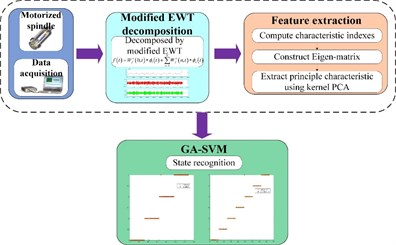The paper is arranged as follows: Section 2 briefly introduces the theoretical background of EWT, Kernel PCA, and SVM, and a new approach to decide the number of the Fourier spectrum segment when using EWT is also proposed. In Section 3, the data acquisition of single and compound faults in a motorized spindle is described in detail. Section 4 presents the data processing and experimental results. Moreover, a comparative study among modified EWT-Kernel PCA, EMD, EEMD, LMD and EWT (the proper number of segment is selected) is executed. Finally, conclusions are drawn in Section 5.

## 2. Methodology

### 2.1.1. Empirical wavelet transform

EWT is a self –adaptive algorithm appropriate for processing non-linear and non-stationary signals by designing a series of bandpass filters. The self-adaptive property mainly embodies the support of filters depends on where the information embedded in the analyzed signal is situated. Signal modes are extracted by EWT, which are AM-FM components that have compact support to the Fourier spectrum . Assuming that the Fourier spectrum $\left[0,\pi \right]$ (frequency $\omega \in \left[0,\pi \right]$) is partitioned into $N$ consecutive segments, which correspond to different modes, ${\omega }_{n}$ is defined as the limit between each segment (where ${\omega }_{0}=0$ and ${\omega }_{n}=\pi$), and the transition phase, which exists centered around each ${\omega }_{n}$, is denoted as ${T}_{n}$ of width $2{\tau }_{n}$. A proportional relationship between ${\omega }_{n}$ and ${\tau }_{n}$ exists, namely ${\omega }_{n}:{\tau }_{n}=\gamma {\omega }_{n}$, where $0<\gamma <1$. The idea used in the construction of both Littlewood-Paley and Meyer’s wavelets is applied on the creation of empirical wavelets. For $\forall n>0$, the empirical scaling function ${\stackrel{^}{\varphi }}_{n}\left(\omega \right)$ and the empirical wavelets ${\stackrel{^}{\psi }}_{n}\left(\omega \right)$ are given by the following equations:

1
${\stackrel{^}{\varphi }}_{n}\left(\omega \right)=\left\{\begin{array}{l}1,\left|\omega \right|\le \left(1-\gamma \right){\omega }_{n},\\ \mathrm{c}\mathrm{o}\mathrm{s}\left[\frac{\pi }{2}\beta \left(\frac{1}{2\gamma {\omega }_{n}}\left(\left|\omega \right|\right)-\left(1-\gamma \right){\omega }_{n}\right)\right],\left(1-\gamma \right){\omega }_{n}\le \left|\omega \right|\le \left(1+\gamma \right){\omega }_{n}\\ 0,\mathrm{}\text{otherwise},\end{array}\right\,$
2
${\stackrel{^}{\psi }}_{n}\left(\omega \right)=\left\{\begin{array}{l}1,\left(1+\gamma \right){\omega }_{n}\le \left|\omega \right|\le \left(1-\gamma \right){\omega }_{n+1},\\ \mathrm{c}\mathrm{o}\mathrm{s}\left[\frac{\pi }{2}\beta \left(\frac{1}{2\gamma {\omega }_{n+1}}\left(\left|\omega \right|-\left(1-\gamma \right){\omega }_{n+1}\right)\right)\right],\left(1-\gamma \right){\omega }_{n+1}\le \left|\omega \right|\le \left(1+\gamma \right){\omega }_{n+1},\\ \mathrm{s}\mathrm{i}\mathrm{n}\left[\frac{\pi }{2}\beta \left(\frac{1}{2\gamma {\omega }_{n}}\left(\left|\omega \right|-\left(1-\gamma \right){\omega }_{n}\right)\right)\right],\left(1-\gamma \right){\omega }_{n}\le \left|\omega \right|\le \left(1+\gamma \right){\omega }_{n},\\ 0,\text{otherwise}.\end{array}\right\$

To obtain a tight frame, parameter $\gamma$ satisfies following condition:

3
$\gamma

The function $\beta \left(x\right)$ is an arbitrary ${C}^{k}\left(\left[0,1\right]\right)$ function, such that:

4
$\beta \left(x\right)=\left\{\begin{array}{l}0,\mathrm{}x\le 0,\beta \left(x\right)+\beta \left(x+1\right)=1,\mathrm{}\mathrm{}\mathrm{}\mathrm{}\forall x\in \left[0,1\right],\\ 1,\mathrm{}\mathrm{}x\ge 1.\end{array}\right\$

Numerous functions satisfy these properties, and the following function is frequently used :

5
$\beta \left(x\right)={x}^{4}\left(35-84x+70{x}^{2}-20{x}^{3}\right).$

Similar to wavelet transform, EWT is denoted as ${W}_{f}^{\epsilon }\left(n,t\right)$. Its detail coefficients are defined by the inner products of raw signals and the empirical wavelets, shown in the following equation:

6
${W}_{f}^{\epsilon }\left(n,t\right)=\int f\left(\tau \right)\overline{{\psi }_{n}\left(\tau -t\right)}d\tau .$

The approximation coefficients are acquired by the inner products of raw signals and the scaling function as in the following equation:

7
${W}_{f}^{\epsilon }\left(0,t\right)=\int f\left(\tau \right)\overline{{\varphi }_{1}\left(\tau -t\right)}d\tau .$

The reconstruction and the empirical mode${f}_{k}$ are obtained by:

8
$f\left(t\right)={W}_{f}^{\epsilon }\left(0,t\right)*{\varphi }_{1}\left(t\right)+\sum _{n=1}^{N}{W}_{f}^{\epsilon }\left(n,t\right)*{\varphi }_{n}\left(t\right),$
9
$\left\{\begin{array}{l}{f}_{0}\left(t\right)={W}_{f}^{\epsilon }\left(0,t\right)*{\varphi }_{1}\left(t\right),\\ {f}_{k}\left(t\right)={W}_{f}^{\epsilon }\left(k,t\right)*{\psi }_{k}\left(t\right),\end{array}\right\$

where $f$ is the signal, $t$ is the time, and $\tau$ is the time variable.

### 2.1.2. Determine the number of the Fourier spectrum segment

In order to find the limits ${\omega }_{n}$ between each segment, the method that computes the local maxima and then the boundaries are set as the smallest minima between consecutive maxima is adopted in this paper. However, the number of segments of the Fourier spectrum is difficult to determine because of lacking priori information for practical analysis. Excessive or less segment contradicts the effect of fault diagnosis. Therefore, determining the proper number of segments is crucial.

Fig. 2Framework of the proposed method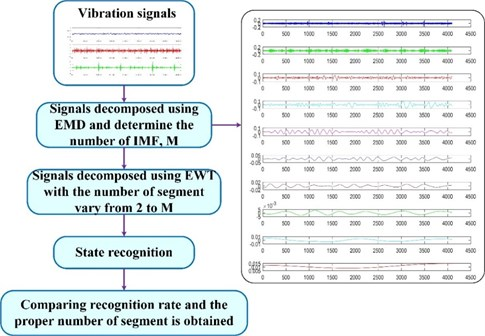EMD is well known in suffering from mode mixing. The proper number of the Fourier spectrum segment is believed to be less than the number of IMF using EMD in this paper, and the IMF number is denoted as $M$. Afterwards, the proper number of segments is easily determined by comparing the recognition rate of the fault diagnosis using GA-SVM with the number of segment vary from 2 to $M$. The framework of the proposed method for determining the optimal number of EWT segments is shown in Fig. 2.

### 2.2. Kernel principal component analysis (Kernel PCA)

Although PCA can perform well in processing a set of linear data, it is not applicable for non-linear data. To deal with non-linear data, the extended version of PCA, KPCA, is introduced . By using the kernel method, the non-linear data can be mapped into a higher dimensional space in which they vary linearly. Given a set of centered data ${x}_{k}$, $k=1,...,M$, ${x}_{k}\in {R}^{d}$, then these centered data are mapped into a higher dimensional feature space $F$ by non-linear mapping $\varphi :{R}^{d}\to F$. Covariance matrix is computed in $F$ as:

10
$\overline{C}=\frac{1}{M}\sum _{j=1}^{M}\varphi \left({x}_{j}\right)\varphi {\left({x}_{j}\right)}^{T}.$

The eigenvalues $\lambda \ge 0$ and eigenvectors $\mathbf{V}$ satisfy the following equation:

11
$\lambda \mathbf{V}=\overline{C}\mathbf{V}=\frac{1}{M}\sum _{j=1}^{M}\left(\varphi \left({x}_{j}\right)\cdot V\right)\varphi \left({x}_{j}\right).$

All solutions $\mathbf{V}$ with $\lambda \ne 0$ lie in the span of $\varphi \left({x}_{1}\right),...\varphi \left({x}_{M}\right)$. Hence, the following equation is obtained:

12
$\left\{\begin{array}{l}\lambda \left(\varphi \left({x}_{k}\right)\cdot \mathbf{V}\right)=\left(\varphi \left({x}_{k}\right)\cdot \overline{C}\mathbf{V}\right),k=1,...,M,\\ \mathbf{V}=\sum _{i=1}^{M}{\alpha }_{i}\varphi \left({x}_{i}\right),\end{array}\right\$

where ${\alpha }_{i}\left(i=1,...,M\right)$ is the coefficient. According to Eq. (12), we can obtain:

13
$\lambda \sum _{i=1}^{M}{\alpha }_{i}\left(\varphi \left({x}_{k}\right)\cdot \varphi \left({x}_{i}\right)\right)=\frac{1}{M}\sum _{i=1}^{M}{\alpha }_{i}\left(\varphi \left({x}_{k}\right)\cdot \sum _{j=1}^{M}\varphi \left({x}_{j}\right)\right)\left(\varphi \left({x}_{j}\right)\cdot \varphi \left({x}_{i}\right)\right).$

The kernel trick ${K}_{ij}=\left(\varphi \left({x}_{i}\right)\cdot \varphi \left({x}_{j}\right)\right)$ is applied, and $\mathbf{K}$ is the $M×M$ kernel matrix. We can obtain:

14
$M\lambda \mathbf{K}\alpha ={\mathbf{K}}^{2}\alpha ,$

where $\alpha$ is the column vector with entries ${\alpha }_{1},...,{\alpha }_{M}$. To find the solutions of Eq. (14), we solve the eigenvalue problem:

15
$M\lambda \alpha =\mathbf{K}\alpha .$

Define ${\lambda }_{1}\le {\lambda }_{2}\le \cdots \le {\lambda }_{M}$ as the eigenvalues of $\mathbf{K}$, ${\alpha }^{1},...,{\alpha }^{M}$, which are the corresponding complete set of eigenvectors, and ${\lambda }_{p}$ is the first non-zero eigenvalue. According to a specific requirement, normalization processing in feature space $F$ is executed for the corresponding eigenvectors ${\alpha }^{1},...,{\alpha }^{M}$ where:

16
$\left({\mathbf{V}}^{k}\cdot {\mathbf{V}}^{k}\right)=1,k=p,...,M.$

By synthesizing Eq. (12) and Eq. (15), the following equation is obtained:

17
$1=\sum _{i,j=1}^{M}{\alpha }_{i}^{k}{\alpha }_{j}^{k}\left(\varphi \left({x}_{i}\right)\cdot \varphi \left({x}_{j}\right)\right)=\sum _{i,j=1}^{M}{\alpha }_{i}^{k}{\alpha }_{j}^{k}{K}_{ij}={\lambda }_{k}\left({\alpha }^{k}\cdot {\alpha }^{k}\right).$

Computation of the projections onto the eigenvectors ${\mathbf{V}}^{k}$ in $F$ is necessary to extract the principal component:

18
$\left({\mathbf{V}}^{k}\cdot \varphi \left(x\right)\right)=\sum _{i=1}^{M}{\alpha }_{i}^{k}\left(\varphi \left({x}_{i}\right)\cdot \varphi \left(x\right)\right).$

In addition, the problem on selecting the proper number of principal components has attracted considerable interests for the past decade. A series of criteria have been adopted in the selection of the number of principal components, and the most frequently used approach, that is, cumulative contribution limit , is applied in this paper.

### 2.3. Support vector machine optimized by genetic algorithm

SVM was first proposed by Vapnik et al. based on statistical learning theory . Through time, SVM become increasingly popular in machine learning, and has been widely used in machine fault diagnosis because of its better generalization and higher recognition rate for analysis of small set of samples compared to traditional pattern recognition approaches such as artificial neural networks (ANN). The basic principle of SVM for typical two-class classification problems is described as follows. Given a set of training data $\left\{{x}_{i},{y}_{i}\right\}$, $1\le i\le Q$ and ${y}_{i}\in \left\{-1,+1\right\}$, SVM aims to find a high dimensional space in which the training data can be separated by the hyperplane as accurately as possible. In the linear separable case, the hyperplane can be expressed in the following equation:

19
$\mathbf{w}\cdot \mathbf{x}+b=0,$

where $\mathbf{w}$ is the $Q$-dimensional vector, $\mathbf{x}$ is the input vector, and $b$ is the scalar.

The training data are classified into two portions, and they are referred as negative and positive objects by hyperplane. The positive and negative object labels are ${y}_{i}=+1$ and ${y}_{i}=-1$, respectively. An optimal separating hyperplane, which creates the maximum distance between two edge lines, depended on support vectors. A linear classification is shown in Fig. 3. The slack variables ${\zeta }_{i}$ and the penalty parameter $C$ are introduced because of considering the noise. Thus, the optimal problem can be expressed as:

20
$\begin{array}{l}\text{minimize}\mathrm{}\frac{1}{2}{‖\mathbf{w}‖}^{2}+C\sum _{i=1}^{N}{\zeta }_{i},\\ \text{subject}\text{}\text{to}\text{}\mathrm{}\left\{\begin{array}{l}{y}_{i}\left({\mathbf{w}}^{T}{x}_{i}+b\right)\ge 1-{\zeta }_{i},\\ {\zeta }_{i}\ge 0,i=1,...,Q,\\ C\ge 0,\end{array}\right\\end{array}$

where $‖\mathbf{w}‖$ is the Euclidean norm of $\mathbf{w}$.

Kernel transform is applied when the original data should be mapped into a higher feature space facing the non-linear problem, and $K$ is the kernel function. The SVM classifier output is then expressed as:

21
$f\left(x\right)=sign\left(\sum _{i=1}^{N}{\mu }_{i}{y}_{i}K\left(\mathbf{x},{\mathbf{x}}_{i}\right)+b\right),$

where ${\mu }_{i}$ is the Lagrange multipliers and ${\mathbf{x}}_{i}$ is the support vector.

Fig. 3Linear classification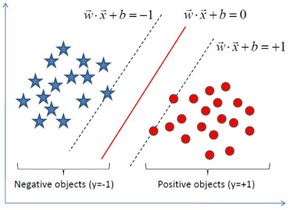Radial basis function is a common kernel function, and it has been used as kernel of SVM in numerous articles. Hence, it is chosen as the kernel function in this study. The radial basis function is defined as follows:

22
$K\left(\mathbf{x},{\mathbf{x}}_{i}\right)=\mathrm{e}\mathrm{x}\mathrm{p}\left(-\gamma {‖x-{x}_{i}‖}^{2}\right).$

In which $\gamma =1/2{\sigma }^{2}$ is the kernel parameter and $\sigma$ is the width parameter of the radical basis function.

It is essential to select a proper combination of parameters for achieving best prediction accuracy in SVM. It is well known that the GA is a global optimization algorithm based on Charles Darwin’s theory of natural selection and evolution, and it is a powerful tool to solve the optimization problem. Therefore, in order to find the best parameter combination, the GA  is applied into SVM. The steps of a simple GA are shown in Fig. 4. In this paper, population size, the number of iterations, crossover probability and mutation probability are set to 20, 200, 0.9 and 0.05, respectively. GA is introduced to find a proper combination of parameters, namely $C$ and $\gamma$, and the value ranges are both set to the range of 0 to 200. In addition, the SVM recognition rate serves as the fitness function. The flowchart of GA-SVM is presented in Fig. 5.

Fig. 4Genetic algorithm flowchart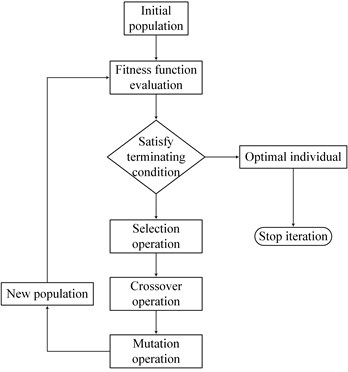## 3. Data acquisition of single and compound faults

According to the statistical analysis, bearing fault contributes the largest proportion, accounting for 44.4 %, in the mechanical spindle of machining center . Thus, execution of bearing fault diagnosis of the motorized spindle is necessary. In addition, rotor unbalance and bolt looseness fault immensely impact the performance of motorized spindle. To verify the effectiveness of the proposed method, the bearing fault data, which comes from the bearing data center of Case Western Reserve University was utilized for single fault diagnosis . As for the compound fault diagnosis, vibration data was acquired in different fault conditions, namely normal, rotor unbalance, bolt looseness, and compound fault (rotor unbalance and bolt looseness coexist).

Fig. 5Flowchart for GA-SVM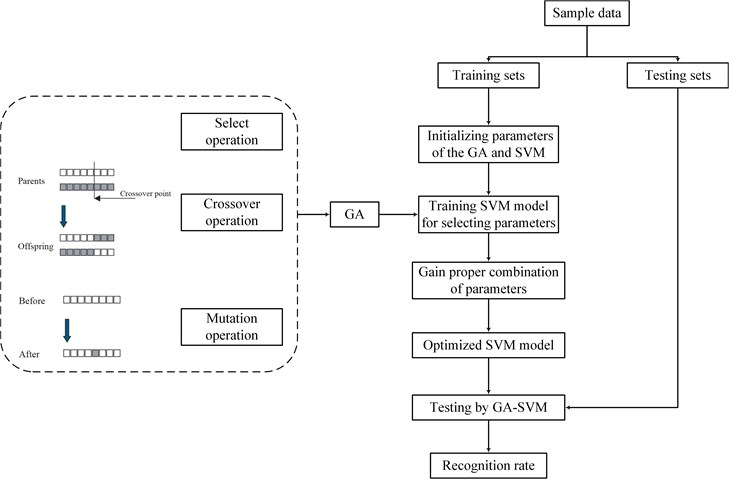### 3.1. Data acquisition of single faults

Experiments for bearing single faults were conducted in a test stand. The test stand is composed of a three-phase induction motor, an AC electrical dynamometer, a torque sensor, and a control system. The 6205-2RS JEM SKF, deep groove ball bearing, was selected as test bearing, which supports the induction motor shaft. The localized defects were created on the inner and outer raceways and the ball by utilizing electro-discharge machining. The diameters of single point faults were 0.1778 and 0.3556 mm, and the depth of defect was consistent at 0.2794 mm. Accelerometers were used to acquire vibration signals, which were fixed in the drive end of the induction motor housing with magnetic bases, in which the laying position was at the 6 o’clock position. The vibration signals were acquired at a sampling frequency of 12000 Hz, and the motor output speed was 1772 rpm with 1 horsepower motor load.

### 3.2. Data acquisition of compound faults

Compound fault experiments in a motorized spindle were conducted in the test platform as shown in Fig. 6.

The test platform consists of a data acquisition system, oil-air lubrication, cooling system, frequency converter, loading rod, and a motorized spindle. The loading rod was installed in the motorized spindle, and the eccentric mass was fixed in the loading rod, which realizes the fault simulation for rotor unbalance. In addition, the motorized spindle was fixed by four bolts, and three of them were loosened when the bolt looseness fault experiment was conducted. Considering safety, the speed of the motorized spindle was set to 5000 rpm. Vibration signals were collected in various states at a sampling frequency of 5000 Hz. Electromagnetic interference should be given attention because of the frequency converter. Therefore, vibration signals were preprocessed using Penalty wavelet threshold de-noising, which can increase SNR.

Fig. 6Test platform of the motorized spindle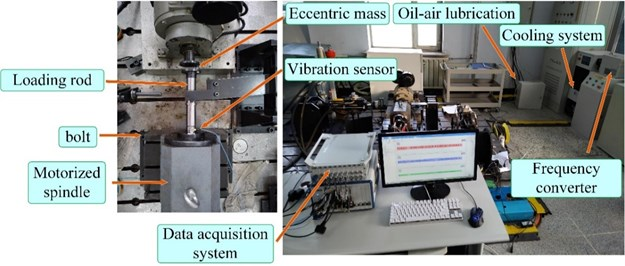## 4. Data processing and experimental results

### 4.1. Single fault diagnosis

In the experiment of single faults, vibration signals are collected in seven states, namely the normal condition, the inner raceway fault (two states with different fault diameters), the ball fault (two states with different fault diameters), and the outer raceway fault (two states with different fault diameters). The fault diameters are 0.1778 mm and 0.3556 mm respectively. The time domain waveforms of vibration signals collected in seven states are presented in Fig. 7. Single fault diagnosis aims to identify the seven states effectively and accurately. Vibration signals collected in a certain state are divided into 29 subsets with equal lengths of 4096. A detailed description for vibration signals is shown in Table 1.

Fig. 7Time domain waveforms of vibration signals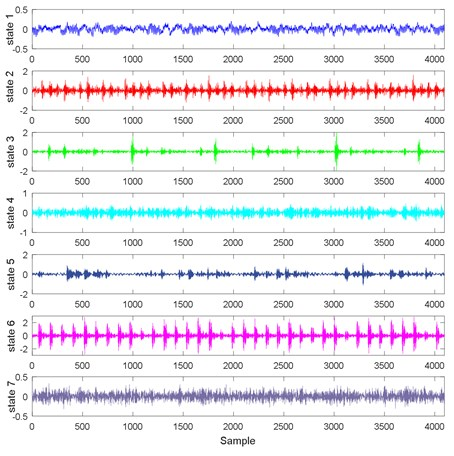The EWT-Kernel PCA method is applied to process vibration signals. To start with, the proper number of segment needs to be determined. EMD is initially applied to analyze vibration signals, and the number of intrinsic mode function obtained using EMD is between 10 and 12. Considering that EMD suffers from the major shortcoming of mode mixing, the determination of the proper number of segment is reasonably not larger than 10. Vibration signals collected at different states are then decomposed using EWT, and the number of segment varies from 2 to 10. Energy, kurtosis, and root mean square are selected as characteristic indexes. Characteristic indexes of the signals, which are decomposed by using EWT, are extracted to construct Eigen-matrix which serves as input of GA-SVM. The recognition rate is obtained using GA-SVM, and the results are presented in Table 2. The proper number of segment is determined by comparing the recognition rate.

Table 1Detailed description of vibration signals

 Class Bearing fault Defect size (mm) Sample number State 1 Normal 0 29 State 2 Inner raceway 0.1778 29 State 3 Inner raceway 0.3556 29 State 4 Ball 0.1778 29 State 5 Ball 0.3556 29 State 6 Outer raceway 0.1778 29 State 7 Outer raceway 0.3556 29

Table 2Recognition rate (single faults)

 Number of segment Recognition rate (%) Time (s) 2 98.8095 24.0550 3 100 25.9561 4 97.6190 26.9161 5 95.2381 27.7974 6 95.2381 32.0490 7 95.2381 32.1671 8 96.4286 32.5506 9 94.0476 33.2683 10 94.0476 33.7320

According to Table 2, the highest recognition rate is easily found when the number of segment varies from 2 to 10. From Table 2, we can see that the highest recognition rate is 100 % when the number of segment is 3; therefore, the proper number of segment is 3. Kernel PCA is applied to extract the principal characteristics from Eigen-matrix. Furthermore, cumulative contribution limit is adopted to determine the number of principal characteristics. The detailed description for cumulative contribution is presented in Table 3.

Table 3Cumulative contribution

 Serial number Eigenvalue Cumulative contribution 1 124.6073 0.6412 2 24.2019 0.7657 3 16.1617 0.8489 4 9.6115 0.8984 5 7.7455 0.9382 6 4.9167 0.9635 7 3.0010 0.9790 8 2.6911 0.9928 9 1.3953 1

The threshold of cumulative contribution limit is set to 0.90 in this paper. According to Table 3, the anterior five characteristics are extracted as principal characteristics. Finally, the new Eigen-matrix serves as the input of GA-SVM. Table 1 shows that a total of 29 samples are obtained in each state, and the anterior 60 % of samples are selected as training samples. For comparison, EMD, EEMD and LMD are applied to decompose vibration signals collected in different states as well. When using EEMD, there are two parameters are needed to be set, which are the ensemble number and the amplitude of the added white noise. In this study, the ensemble number and the amplitude of the added white noise are set to 100 and 0.2, respectively . The results of EEMD/EWT/LMD decomposition are given in Figs. 8-13. In this paper, we only give the results of two states, namely State 1 (Normal) and State 7 (Outer raceway fault, the defect size is 0.3556 mm) because of limited space. Then energy, kurtosis, and root mean square are computed to construct an Eigen-matrix, which is inputted in GA-SVM, and the result is shown in Figs. 14-17. A detailed comparison results for single fault diagnosis is presented in Table 4. According to Table 4, modified EWT-Kernel PCA, EWT ($N=$ 3), EEMD and LMD are superior to EMD in two aspects: recognition rate and computing time. In addition, modified EWT–Kernel PCA, EWT ($N=$ 3), EEMD and LMD can reach a high recognition rate. As for computing time, the modified EWT-Kernel PCA is better than EWT ($N=$ 3), EEMD and LMD.

Fig. 8The signal decomposition of State 1 by EEMD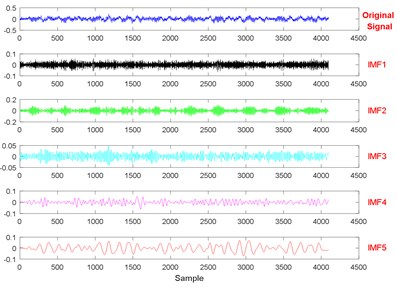a)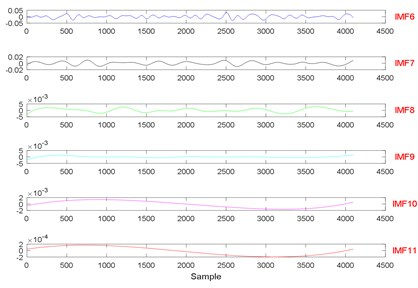b)

Fig. 9The signal decomposition of State 7 by EEMD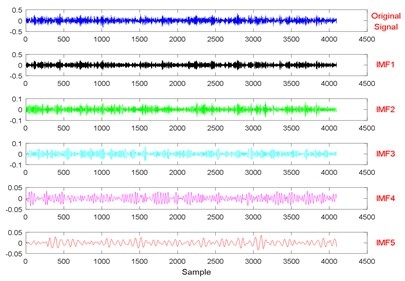a)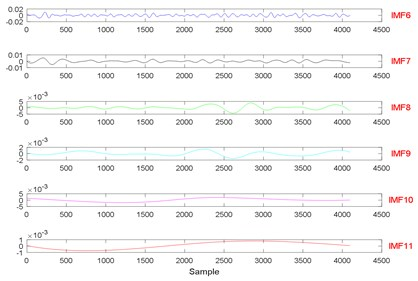b)

Fig. 10The signal decomposition of State 1 by EWT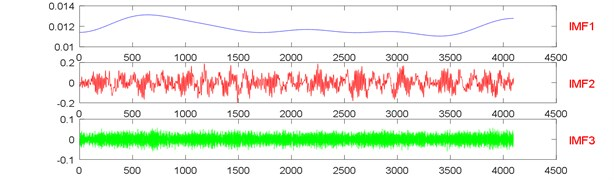Fig. 11The signal decomposition of State 7 by EWT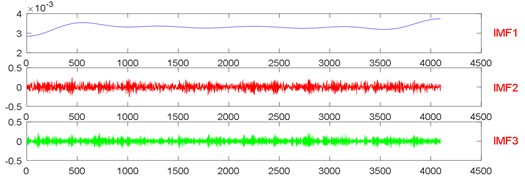Fig. 12The signal decomposition of State 1 by LMD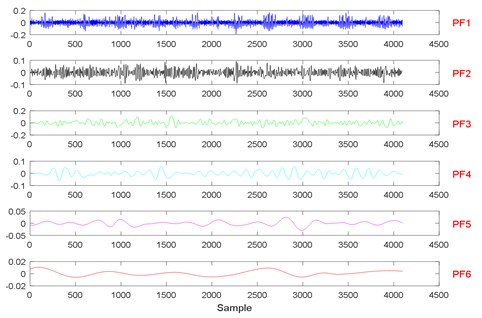Fig. 13The signal decomposition of State 7 by LMD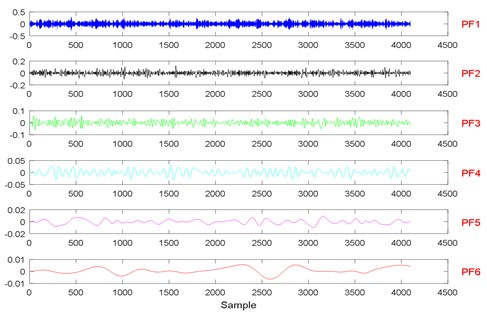Fig. 14State recognition using the modified EWT-Kernel PCA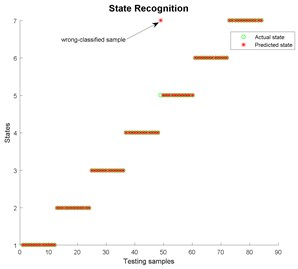Fig. 15State recognition using EMD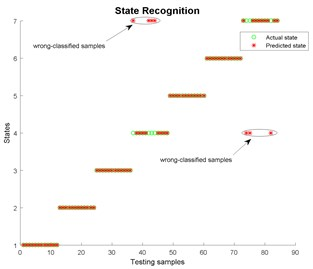Fig. 16State recognition using EEMD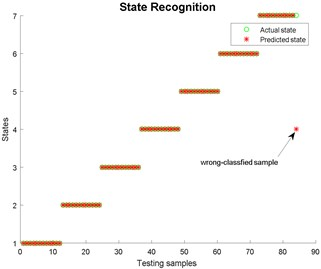Fig. 17State recognition using LMD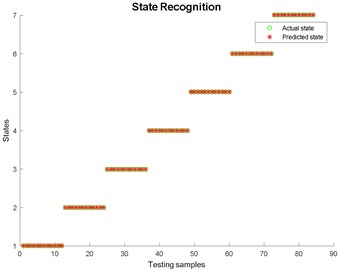Table 4Comparison results (single fault diagnosis)

 Method Recognition rate (%) Time (s) Optimum parameters $C$ $\gamma$ Modified EWT-Kernel PCA 98.8095 20.8028 2.9676 18.6115 EWT ($N=$ 3) 100 25.9561 21.5076 1.9846 EMD 91.6667 32.4293 3.2776 0.7719 EEMD 98.8095 27.3467 13.4472 0.4829 LMD 100 27.9825 6.8211 1.0040

### 4.2. Compound fault diagnosis

In the compound fault experiment, vibration signals are collected in four states, namely normal, rotor unbalance, bolt looseness, and compound fault (rotor unbalance and bolt looseness coexist). The time domain waveforms of vibration signals, which are preprocessed using Penalty wavelet threshold de-noising, are presented in Fig. 18.

To identify the four states effectively and accurately, the preprocessed vibration signals are segmented into 80 subsets of equal lengths of 4096. Further description is presented in Table 5.

Fig. 18Time domain waveform of vibration signals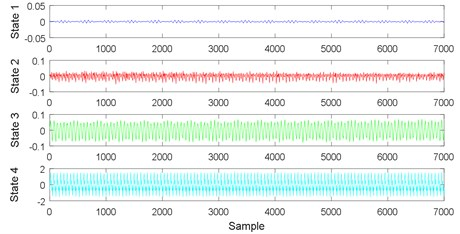Table 5Further description of the preprocessed vibration signals

 Class Fault Sample number State 1 Normal 80 State 2 Bolt looseness 80 State 3 Rotor unbalance 80 State 4 Compound fault 80

The processing procedures of compound fault diagnosis are the same as that of single fault diagnosis. EMD is initially utilized to analyze vibration signals, and the number of intrinsic mode function obtained using EMD is between 9 and 11. The proper number of segment is considered less than 9 because EMD suffers from mode mixing. Vibration signals collected in four states are then decomposed using EWT, and the number of segment varies from 2 to 9. Energy, kurtosis and root mean square are extracted to construct Eigen-matrix, which serves as the input of GA-SVM. The results are presented in Table 6. The comparison results of the recognition rates of compound fault diagnosis revealed that the proper number of segment can be found.

Table 6Recognition rate (compound faults)

 Number of segment Recognition rate (%) Time (s) 2 49.2188 24.6227 3 75.7813 26.6460 4 88.2813 26.4702 5 97.6563 25.1563 6 78.1250 27.3122 7 95.3125 24.1459 8 96.0938 26.7265 9 81.2500 28.1158

Table 6 shows that the highest recognition rate is obtained when the number of segment varies from 2 to 9. Hence, the proper number of segment is 5. Kernel PCA is applied to extract principal characteristics from Eigen-matrix. Cumulative contribution limit is adopted to determine the number of principal characteristics. The detailed description for cumulative contribution is presented in Table 7.

Table 7Cumulative contribution

 Serial number Eigenvalue Cumulative contribution 1 119.2375 0.5610 2 14.9248 0.6312 3 14.0555 0.6973 4 11.8911 0.7533 5 9.2964 0.7970 6 7.0838 0.8303 7 6.5162 0.8610 8 5.6663 0.8877 9 4.9138 0.9108 10 4.3771 0.9314 11 3.9011 0.9497 12 2.9933 0.9638 13 2.7913 0.9769 14 2.5411 0.9889 15 2.3607 1

According to Table 7, because the threshold of the cumulative contribution limit is set to 0.90 in this paper, the anterior nine characteristics are extracted as principal characteristics. Finally, the new Eigen-matrix serves as the input of GA-SVM. Based on Table 5, a total of 80 samples are obtained in each state, and the anterior 60 % of samples are selected as training samples. The results of EEMD/EWT/LMD decomposition are given in Figs. 19-24. In this paper, we only give the results of two states, namely State 1 (Normal) and State 4 (Compound fault) because of limited space. The results of state recognition using modified EWT-Kernel PCA, EMD, EEMD and LMD are shown in Figs. 25-28. The result of the detailed comparison of compound fault diagnosis is presented in Table 8. According to Table 8, modified EWT-Kernel PCA, EWT ($N=$ 5) EEMD and LMD are obviously far superior to EMD in terms of recognition rate. For computing time, the modified EWT-Kernel PCA is better than EWT ($N=$ 5), EMD, EEMD and LMD.

Table 8Comparison results (compound faults diagnosis)

 Method Recognition rate (%) Time (s) Optimum parameters $C$ $\gamma$ Modified EWT-Kernel PCA 98.4375 18.0463 104.7613 1.5739 EWT ($N=$ 5) 97.6563 25.1563 87.7794 1.8892 EMD 94.5313 43.0471 46.9961 0.9804 EEMD 99.2188 43.5325 100.1085 0.8619 LMD 99.2188 20.2320 9.3590 0.5783

Fig. 19The signal decomposition of State 1 by EEMD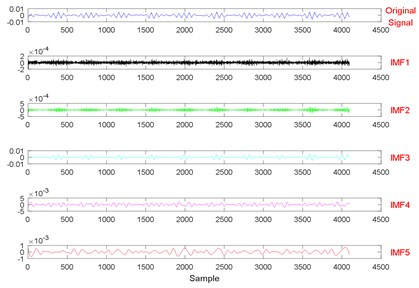a)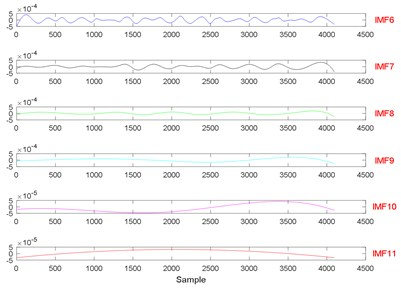b)

Fig. 20The signal decomposition of State 4 by EEMD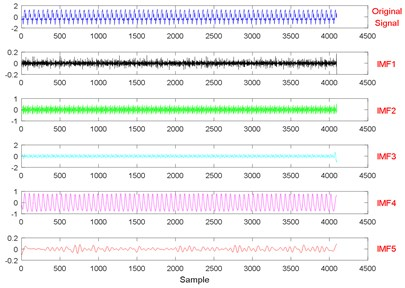a)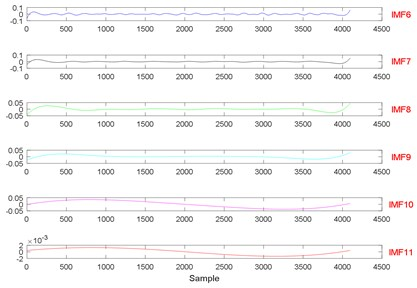b)

Fig. 21The signal decomposition of State 1 by EWT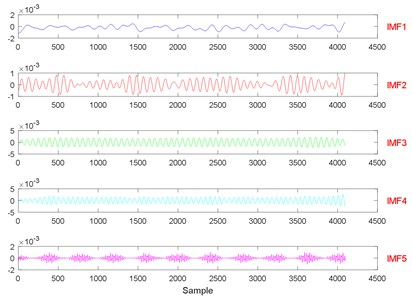Fig. 22The signal decomposition of State 4 by EWT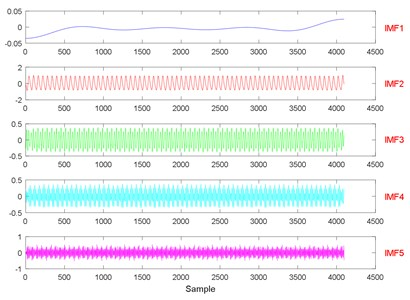Fig. 23The signal decomposition of State 1 by LMD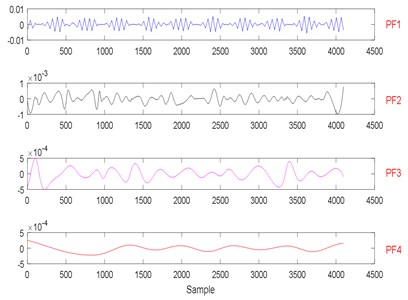Fig. 24The signal decomposition of State 4 by LMD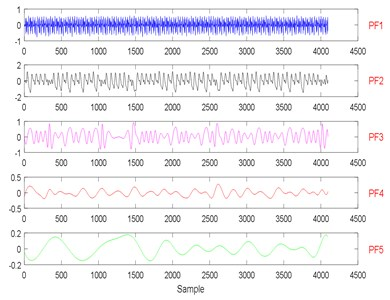Fig. 25State recognition using modified EWT-Kernel PCA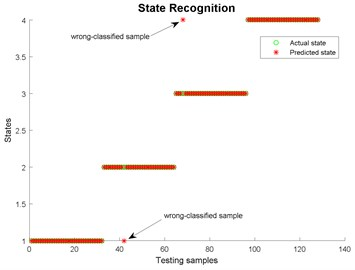Fig. 26State recognition using EMD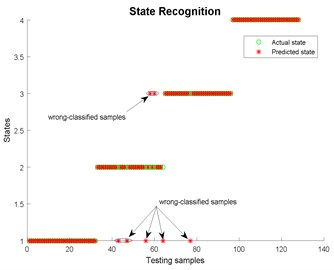Fig. 27State recognition using EEMD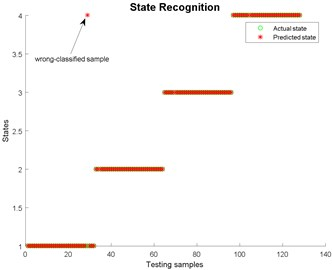Fig. 28State recognition using LMD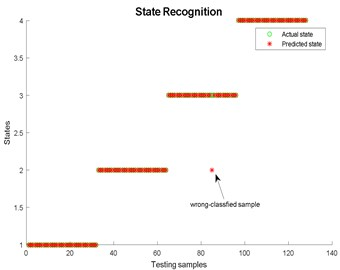## 5. Conclusions

In this paper, a method based on modified EWT and Kernel PCA for the single and compound fault diagnoses of the motorized spindle is investigated. A comparison study among EWT (the proper number of segment is selected), EMD, EEMD, LMD and modified EWT-Kernel PCA is executed. The experimental data obtained from the bearing data center of Case Western Reserve University are utilized for the single fault diagnosis of a motorized spindle, and as for compound fault diagnosis, experiments are carried out on a test platform for a motorized spindle. The analysis results indicate that the proposed method can effectively and correctly performs fault diagnosis. The recognition rate using modified EWT-Kernel PCA reach 98.8095 % and 98.4375 % in terms of single and compound fault diagnoses, respectively, which are higher than those in EMD. Moreover, for single fault diagnosis, the recognition rate using modified EWT-Kernel PCA, EWT, EEMD and LMD are almost the same, and as for compound fault diagnosis, modified EWT-Kernel PCA, EEMD and LMD are superior to EWT ($N=$ 5) and EMD. Compared with EWT, EMD, EEMD and LMD in the aspect of computing time, the proposed method saved 19 %, 35 %, 24 % and 26 % for single fault diagnosis, respectively. For compound fault diagnosis, the proposed method saved 39 %, 58 %, 59 % and 11 %, respectively. Finally, this method can be generalized for another mechanical fault diagnosis.

30 January 2017
Accepted
27 April 2017
Published
30 June 2017
SUBJECTS
Fault diagnosis based on vibration signal analysis
Keywords
motorized spindle
fault diagnosis
modified empirical wavelet transform (EWT)
kernel principal components analysis (Kernel PCA)
Acknowledgements

This work is supported by the Project of Jilin Province, Science and Technology Development Plan of the Fault Diagnosis and Condition Monitoring for Motorized Spindle of Machining Center (Grant No. 20160101278JC) and the Subject of the Research and Application of Rapid Test Technology for Reliability of CNC (Grant No. 2016ZX04004-004) and the Project of the Graduate Innovation Fund of Jilin University (Grant No. 2016027).

Author Contributions

Fei Chen conceived the idea of using modified empirical wavelet transform to analyze vibration data. Chao Chen applied kernel principal component analysis into reducing dimension and guide the writing of the whole paper. Yifeng Ye conducted the method to better diagnosis the single and compound fault of the motorized spindle and wrote the majority of the paper. Weizheng Chen Carried out the experiments. Binbin Xu processed the experimental data and analyzed the results. Zhaojun Yang edited the manuscript and checked the grammatical and spelling errors.